Courses

# Test: Dimensions Of Unit Cells

## 10 Questions MCQ Test Chemistry Class 12 | Test: Dimensions Of Unit Cells

Description
This mock test of Test: Dimensions Of Unit Cells for Class 12 helps you for every Class 12 entrance exam. This contains 10 Multiple Choice Questions for Class 12 Test: Dimensions Of Unit Cells (mcq) to study with solutions a complete question bank. The solved questions answers in this Test: Dimensions Of Unit Cells quiz give you a good mix of easy questions and tough questions. Class 12 students definitely take this Test: Dimensions Of Unit Cells exercise for a better result in the exam. You can find other Test: Dimensions Of Unit Cells extra questions, long questions & short questions for Class 12 on EduRev as well by searching above.
QUESTION: 1

### If an element crystallizes as a simple cube, what is the volume of an element provided its density is 1.5 g/cm3 and atomic mass of the element is 63?

Solution:

Simple cube has 1 atom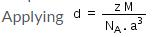Therefore volume of cube i.e. a3 = 6.97 x 10-23 cm3

QUESTION: 2

### Mass of an atom in an unit cell is calculated by which of the following formula if M is the molar mass:

Solution:

(Molar mass = M) (1 molar of atom = M 6.023×1023)( atom = M/Na)

QUESTION: 3

### In the formula to calculate the density of a unit cell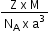what is z ?

Solution:

The correct answer is Option B.
Mass of unit cell = number of atoms in unit cell × mass of each atom = z × m
Where, z = number of atoms in unit cell,
m = Mass of each atom
Mass of an atom can be given with the help of Avogadro number and molar mass as: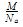Where, M = molar mass
Volume of unit cell, V = a3
⇒ Density of unit cell =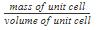⇒ Density of unit cell =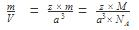QUESTION: 4

Volume of a body centred cubic unit cell is

Solution:

In a body centered cubic unit cell ,√3a = 4r a= 4r/√3 where a = side of the cube volume = a3 = (4r/√3)3.

QUESTION: 5

Density of a unit cell is equal to mass of unit cell divided by

Solution:

Volume of unit cell, V = a3

=> Density of unit cell = mass of unit cell /volume of unit cell

QUESTION: 6

Length a in unit cell is along

Solution:

The correct answer is option A
Unit cell is essentially considered a cube, so a cube has 3 axes. X,Y,Z so X represents length , Y represents breadth, Z represents height.

QUESTION: 7

The number of atoms (z) in simple cubic unit cell is

Solution:

The face-centered cubic (fcc) has a coordination number of 12 and contains 4 atoms per unit cell. The body-centered cubic (bcc) has a coordination number of 8 and contains 2 atoms per unit cell. The simple cubic has a coordination number of 6 and contains 1 atom per unit cell.

QUESTION: 8

X-ray diffraction studies show that copper crystallizes in an fcc unit cell with cell edge of 3.608 x 10-8 cm. In a separate experiment, copper is determined to have a density of 8.92 g/cm3, calculate the atomic mass of copper.

Solution:

In case of fcc lattice,number of atoms per unit cell,x=4 atoms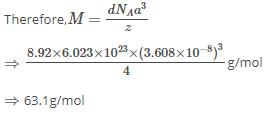∴  Atomic mass of copper =63.1u

QUESTION: 9

An element with bcc geometry has atomic mass 50 and edge length 290 pm. The density of unit cell will be

Solution:

Length of the edge , a = 290 pm =290 x 10-10 cm
Volume of unit cell = ( 290 x 10-10 cm )3 = 24.39 x 10-24 cm3

Since it is bcc arrangement,
Number of atoms in the unit cell, Z = 2
Atomic mass of the element = 50

Mass of the atom = atomic mass/ Avogadro number = M/No = 50/6.02 x 1023

Mass of the unit cell = Z x M/No = 2 x 50/6.02 x 1023 = 100/6.23 x 1023
Therefore , density = mass of unit cell / volume of unit cell
= 100/6.023 x 1023 x 24.39 x 10-24 = 6.81 g cm-3

QUESTION: 10

The number of octahedral voids (s) per atom present in a cubic close-packed structure is

Solution:

No of atoms per unit cell is 4,,it means it contain 4 octahedral voids and 8 tetrahedral voids

Explanation:

Total no of octahedral voids per atom is 1